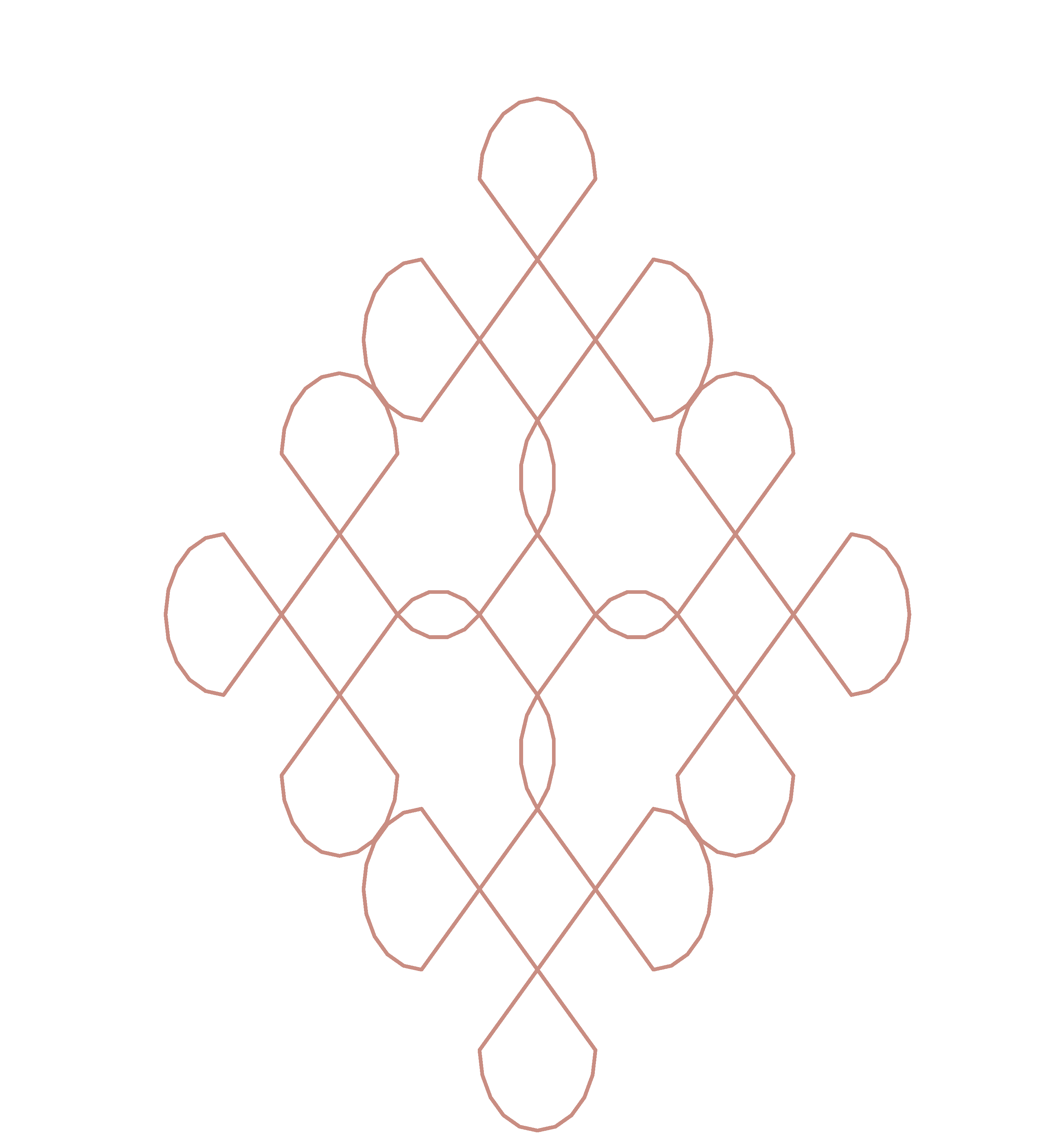# Procedural art: Rangolis

(02 Mar 2019) python art

The `turtle` package in python makes generating procedural art trivial. I really want to explore L-systems and fractals. I was thinking of space filing patterns and I came across a link to kolams or rangolis on wikipedia.

Question: Can I make the (loop-line-)x3(curve-line)x2 pattern?

Surprisingly, this took less than 45 minutes from inspiration to execution.

``````from turtle import *
import numpy as np
``````

Define the loop, and then the rule

``````granularity = 10
diameter = float(100)
straight = diameter/np.sqrt(2.0)
dela = 10
def loop():
delay(dela)
forward(straight)
left(45)
circle(diameter/2,180)
left(45)
delay(dela)
forward(straight)
def rule():
for _ in range(3):
loop()
forward(straight)
for _ in range(2):
#right(45)
circle(diameter/2,90)
forward(straight)
setworldcoordinates(-400,-500,500,200)
pensize(3)
bgcolor("#302c2b")
pencolor("#c98d82")
#pencolor("#e8dcda")